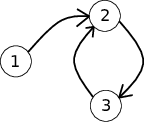• 85通过
• 113提交
• 题目来源
• 评测方式 RemoteJudge
• 标签
• 难度 普及-
• 时空限制 1000ms / 256MB
• 提示：收藏到任务计划后，可在首页查看。

## 推荐的相关题目 显示

本题征求翻译。如果你能提供翻译或者题意简述，请 提交翻译 ，感谢你的贡献。

## 题目描述

In Summer Informatics School, if a student doesn't behave well, teachers make a hole in his badge. And today one of the teachers caught a group of $n$ students doing yet another trick.

Let's assume that all these students are numbered from $1$ to $n$ . The teacher came to student $a$ and put a hole in his badge. The student, however, claimed that the main culprit is some other student $p_a$ .

After that, the teacher came to student $p_a$ and made a hole in his badge as well. The student in reply said that the main culprit was student $p_{p_a}$ .

This process went on for a while, but, since the number of students was finite, eventually the teacher came to the student, who already had a hole in his badge.

After that, the teacher put a second hole in the student's badge and decided that he is done with this process, and went to the sauna.

You don't know the first student who was caught by the teacher. However, you know all the numbers $p_i$ . Your task is to find out for every student $a$ , who would be the student with two holes in the badge if the first caught student was $a$ .

## 输入输出格式

输入格式：

The first line of the input contains the only integer $n$ ( $1 \le n \le 1000$ ) — the number of the naughty students.

The second line contains $n$ integers $p_1$ , ..., $p_n$ ( $1 \le p_i \le n$ ), where $p_i$ indicates the student who was reported to the teacher by student $i$ .

输出格式：

For every student $a$ from $1$ to $n$ print which student would receive two holes in the badge, if $a$ was the first student caught by the teacher.

## 输入输出样例

输入样例#1： 复制
3
2 3 2

输出样例#1： 复制
2 2 3

输入样例#2： 复制
3
1 2 3

输出样例#2： 复制
1 2 3


## 说明

The picture corresponds to the first example test case.When $a = 1$ , the teacher comes to students $1$ , $2$ , $3$ , $2$ , in this order, and the student $2$ is the one who receives a second hole in his badge.

When $a = 2$ , the teacher comes to students $2$ , $3$ , $2$ , and the student $2$ gets a second hole in his badge. When $a = 3$ , the teacher will visit students $3$ , $2$ , $3$ with student $3$ getting a second hole in his badge.

For the second example test case it's clear that no matter with whom the teacher starts, that student would be the one who gets the second hole in his badge.

提示
标程仅供做题后或实在无思路时参考。
请自觉、自律地使用该功能并请对自己的学习负责。
如果发现恶意抄袭标程，将按照I类违反进行处理。